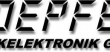Регион:# Doepfer A-137-1 Wave Multiplier I

Код товара: 43858
Гарантия: 12 месяцев
Статус:
Проверка информации со складов...
Wave multiplier

A-137-1 is a voltage controlled waveform multiplier. The basic idea of a wave multiplier is to multiply the waveform of an incoming audio signal (e.g. triangle/sinus/sawtooth of a VCO) within one period. This creates additional harmonics in the audio signal. The period duration and thus also the pitch of the signal remain unchanged - in contrast to frequency multiplication such as with the PLL module A-196.

The A-137-1 works as an "inverse low-pass filter", i.e. it adds additional harmonics to a signal poor in overtones, whereas a low-pass filter (e.g. A-120) filters out overtones from a signal rich in overtones. Therefore, the A-137 gives the best results in combination with comparatively low harmonic waveforms (e.g. triangle, sine) as input signal. For overtone-rich input signals (e.g. sawtooth) the A-137 can also be used, but the effect is not as spectacular as with a triangle signal. With square-wave signals, the effect of the A-137 is hardly noticeable.

The Multiples parameter (adjustable manually and by control voltage) defines the number of waveform multiplications within a period. The two parameters Folding Level and Symmetry (both adjustable manually and by control voltage) set the upper and lower level at which the convolution of the signal takes place. The function of these two parameters is shown graphically in the diagram below. The Harmonics parameter (adjustable manually and by control voltage) adds further harmonics (i.e. in addition to the harmonics created by waveform multiplication) by sharpening the waveform edges (amplification and limiting) and adding overshoot peaks (attenuated waves at the edges).

• Input Level to adjust different input levels for the optimal effect of the module
• Multiples: Number of curve multiplications, manual setting
• Folding Level: Value of the upper and lower folding level, for explanation see sketch below, manual setting
• Symmetry: Symmetry of upper and lower convolution level, see sketch below for explanation, manual adjustment
• Width: 14 TE / HP
• Depth: 65 mm
• Current consumption: +40 mA (+12 V) / -20 mA (-12 V)

Inputs/outputs:

• Audio In - also suitable for control voltages
• Multiples CV In: Control voltage input for the number of curve multiplications, with attenuator
• Harmonics CV In: Control voltage input for the Harmonics parameter, with attenuator
• Folding Level CV In: Control voltage input for the convolution level, with attenuator
• Symmetry CV In: Control voltage input for convolutional symmetry, with attenuator
• Audio Out: Output for the processed audio or control signal.

Оставьте свой номер телефона, менеджер свяжется с вами в ближайшее время.